Refer to our Texas Go Math Grade 3 Answer Key Pdf to score good marks in the exams. Test yourself by practicing the problems from Texas Go Math Grade 3 Module 17 Assessment Answer Key.

Vocabulary

Choose the best term from the box.

Vocabulary
area
perimeter

Question 1.
The distance around a figure is the ___________ . (p. 545)
The distance around the figure is called as perimeter.

Concepts and Skills

Find the perimeter of the figure. Each unit is 1 centimeter. (TEKS 3.7.B)

Question 2.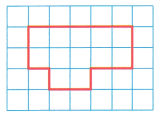____________ centimetersExplanation:
Perimeter the given figure is 15 units
As 1 unit is equal to 1 cm
The perimeter of the given figure is 15 centimeters.

Texas Go Math Grade 3 Answer Key Pdf Module 17 Question 3.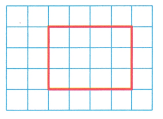____________ centimetersExplanation:
Perimeter the given figure is 14 units
As 1 unit is equal to 1 cm
The perimeter fo the given figure is 14 centimeters.

Find the unknown side lengths.

Question 4.
Perimeter = 33 centimetersg = __________ centimeters
g = 3 cm

Explanation:
The perimeter is 33 centimeters
The sum of the sides I know is 30 centimeters
A related subtraction equation is
g = 33-30
So, the length of side g is 3 centimeters.

Question 5.
Perimeter = 32 feetk = ___________ feet
k = 4 feet

Explanation:

Explanation:
We know that the perimeter of the rectangle is 32 feet
The sum of the lengths of the sides I know is 12+12=24
Subtract the lengths from the perimeter, 32-24=8
Divide the difference by 2
8 ÷  2 = 4
The length of the other side is 4 feet
Therefore, k=4 feet

Use a centimeter ruler to find the perimeter. (TEKS 3.7.B)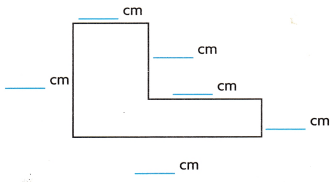___________ centimeters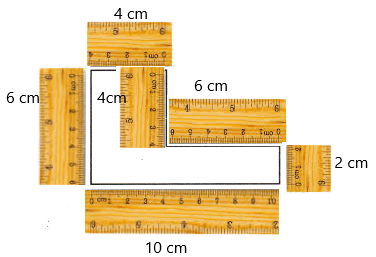Explanation:
Add the lengths of all the sides to find the perimeter
10+6+4+6+2+4=32
Therefore, the perimeter of the given figure is 32 centimeters.

Fill in the bubble for the correct answer choice.

Question 7.
Jacob built a toolbox with a perimeter of 70 inches. What is the length of side m? (TEKS 3..7.B)(A) 50 inches
(B) 20 inches
(C) 25 inches
(D) 10 inches
C. 25 inches

Explanation:
We know that perimeter of the rectangle is 70 inches
The sum of lengths of the sides i know is 10+10=20
Subtract the lengths from the perimeter, 70-20=50
Divide the difference by 2
50 ÷  2 = 25
The length of the other side is 25 inches
Therefore, m = 25 inches

Adrienne is decorating a square picture frame. She glued 32 inches of ribbon around the edge of the frame. What is the length of each side of the picture frame? (TEKS 3.7.B)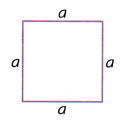(A) 4 inches
(B) 8 inches
(C) 28 inches
(D) 16 inches
B. 8 inches

Explanation:
Adrienne is decorating a square picture frame.
She glued 32 inches of ribbon around the edge of the frame
So, perimeter of picture frame is 32 inches
Perimeter of a square is 4 x length of side
So, 4 x a = 32 then a = 32÷  4
a=8
Therefore, the length of each side of the picture frame is 8 inches.

Question 9.
What is the perimeter of the figure? Each unit is 1 centimeter. (TEKS 3.7.B)Explanation:
Perimeter the given figure is 18 units
As 1 unit is equal to 1 cm
The perimeter fo the given figure is 18 centimeters.

Question 10.
Jeff is making a poster for a car wash for the Campout Club. What is the perimeter of the poster? (TEKS 3.7.B)(A) 8 feet
(B) 3 feet
(C) 6 feet
(D) 4 feet
A. 8 feet

Explanation:
Jeff is making a poster for a car wash for the Campout Club
Perimeter fo a figure is sum of all its sides
The sum of the sides of the poster is 1+3+1+3=8 feet
therefore, Perimeter of the poster is 8 feet.

Go Math Module 17 Assessment Grade 3 Pdf Question 11.
A rectangle has side lengths of 8 inches and 10 inches. What is the perimeter of the rectangle? (TEKS 3.7.B)
(A) 28 inches
(B) 18 inches
(C) 32 inches
(D) 36 inches